# Introduction to the Apriori algorithm (with Java code)

This blog post provides an introduction to the Apriori algorithm, a classic data mining algorithm for the problem of frequent itemset mining. Although Apriori was introduced in 1993, more than 20 years ago, Apriori remains one of the most important data mining algorithms, not because it is the fastest, but because it has influenced the development of many other algorithms.The problem of frequent itemset mining

The Apriori algorithm is designed to solve the problem of frequent itemset mining. I will first explain  this problem with an example. Consider a retail store selling some products. To keep the example simple, we will consider that the retail store is only selling five types of products: I= {pasta, lemon, bread, orange, cake}.   We will call these products “items”.

Now, assume that the retail store has a database of customer transactions: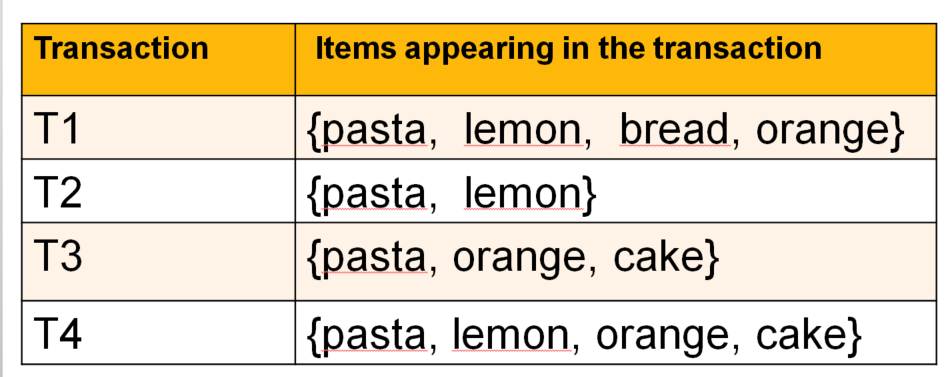This database  contains four transactions. Each transaction is a set of items purchased by a customer (an itemset).  For example, the first transaction contains the items pasta, lemon, bread and orange, while the second transaction contains the items pasta and lemon. Moreover, note that each transaction has a name called its transaction identifier. For example, the transaction identifiers of the four transactions depicted above are T1, T2, T3 and T4, respectively.

The problem of frequent itemset mining is defined as follows. To discover frequent itemsets, the user must provide a transaction database (as in this example) and must set a parameter called the minimum support threshold (abbreviated as minsup).  This parameter represents the number of transactions that an itemset should at least appear in to be considered a frequent itemset and be shown to the user. I will explain this with a simple example.

Let’s say  that the user sets the minsup parameter to two transactions (minsup = 2 ). This means that the user wants to find all sets of items that are purchased together in at least two transactions. Those sets of items are called frequent itemsets. Thus, for the above transaction database,  the answer to this problem is the following set of frequent itemsets:

{lemon}, {pasta}, {orange}, {cake}, {lemon, pasta}, {lemon, orange}, {pasta, orange}, {pasta, cake}, {orange, cake}, {lemon, pasta, orange}

All these itemsets are considered to be frequent itemsets because they appear in at least two transactions from the transaction database.

Now let’s be a little bit more formal. How many times an itemset is bought is called the support of the itemset. For example, the support of {pasta, lemon} is said to be 3 since it is appears in three transactions. Note that the support can also be expressed as a percentage. For example, the support of {pasta, lemon} could be said to be 75% since pasta and lemon appear together in 3 out of 4 transactions (75 % percent of the transactions in the database).

Formally, when the support is expressed as a percentage, it is called a relative support, and when it is expressed as a number of transactions, it is called an absolute support.

Thus, the goal of frequent itemset mining is to find the sets of items that are frequently purchased in a customer transaction  database (the frequent itemsets).

Applications of frequent itemset mining

Frequent itemset mining is an interesting problem because it has applications in many domains. Although, the example of a retail store is used in this blog post, itemset mining is not restricted to analyzing customer transaction databases. It can be applied to all kind of data from biological data to text data. The concept of transactions is quite general and can be viewed simply as a set of symbols. For example, if we want to apply frequent itemset mining to text documents, we could consider each word as an item, and each sentence as a transaction. A transaction database would then be a set of sentences from a text, and a frequent itemset would be a set of words appearing in many sentences.

The problem of frequent itemset mining is difficult

Another reason why the problem of frequent itemset mining is interesting is that it is a difficult problem. The naive approach to solve the problem of itemset mining is to count the support of all possible itemsets and then output those that are frequent. This can be done easily for a small database as in the above example.  In the above example, we only consider five items  (pasta, lemon, bread, orange, cake). For five items, there are 32 possible itemsets. I will show this with a picture: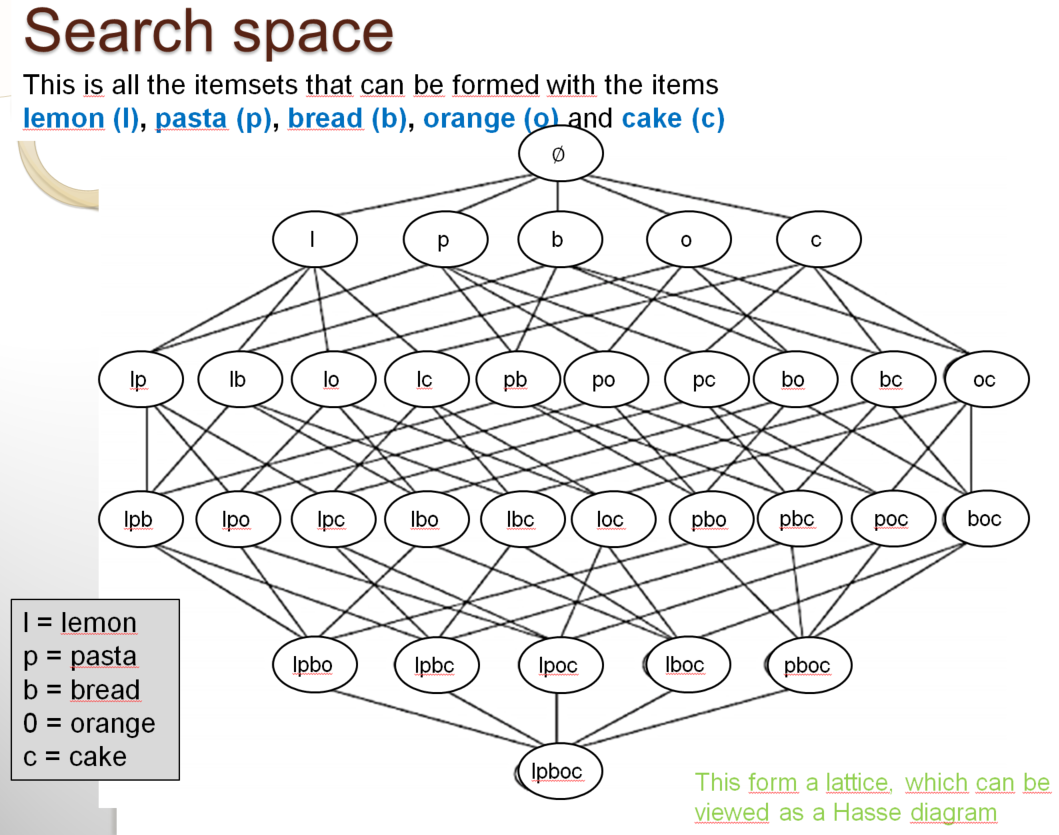In the above picture, you can see all the sets of items that can be formed by using the five items from the example. These itemsets are represented as a Hasse diagram.  Among all these itemsets, the following itemsets highlighted in yellow are the frequent itemsets:Now, a good question is: how can we write a computer program to quickly find the frequent itemsets in a database? In the example, there are only 32 possible itemsets. Thus, a simple approach is to write a program that calculate the support of each itemset by scanning the database. Then, the program would output the itemsets having a support no less than the minsup threshold to the user as the frequent itemsets.  This would work but it would be highly inefficient for large databases. The reason is the following.

In general, if a transaction database has items, there will be  2^x possible itemsets (2 to the power of x).  For example, in our case, if we have 5 items, there are 2^5 = 32 possible itemsets. This is not a lot because the database is small. But consider a retail store having 1,000 items. Then the number of possible itemsets would be:  2^1000 = 1.26 E30, which is huge, and it would simply not be possible to use a naive approach to find the frequent itemsets.

Thus, the search space for the problem of frequent itemset mining is very large, especially if there are many itemsets and many transactions. If we want to find the frequent itemsets in a real-life database, we thus need to design some fast algorithm that will not have to test all the possible itemsets.  The Apriori alorithm was designed to solve this problem.

The Apriori algorithm

The Apriori algorithm is the first algorithm for frequent itemset mining. Currently, there exists many algorithms that are more efficient than Apriori. However, Apriori remains an important algorithm as it has introduced several key ideas used in many other pattern mining algorithms thereafter. Moreover, Apriori has been extended in many different ways and used for many applications.

Before explaining the Apriori algorithm, I will introduce two important properties.

Two important properties

The Apriori algorithms is based on two important properties for reducing the search space. The first one is called the Apriori property (also called anti-monotonicity property). The idea is the following. Let there be two itemsets X and Y such that X is a subset of Y.  The support of Y must be less than or equal to the support of X.  In other words, if we have two sets of items  X and Y such that X is included in Y,  the number of transactions containing Y must be the same or less than the number of transactions containing X.  Let me show you this with an example: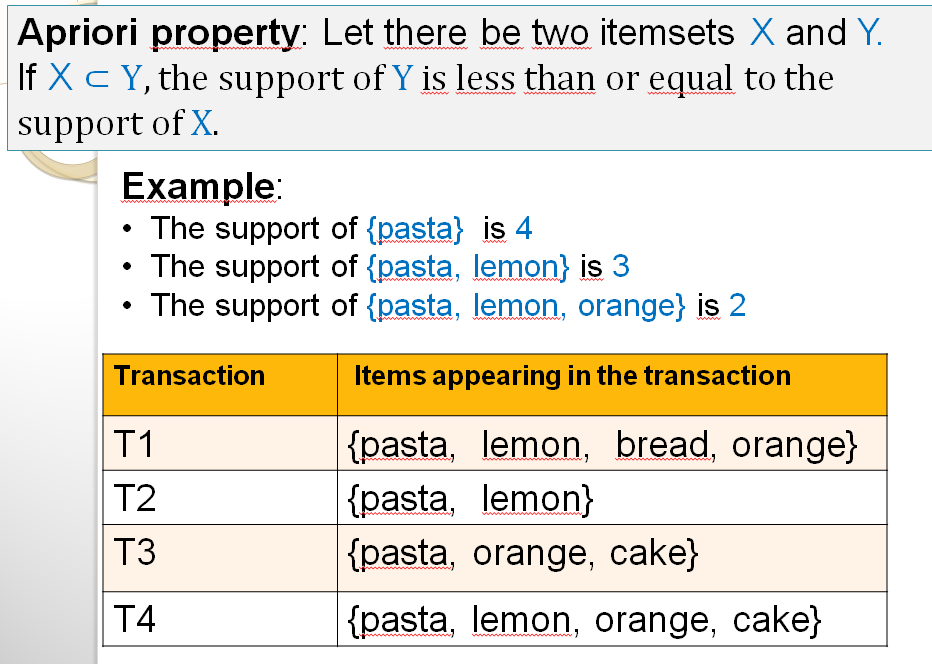As you can see above, the itemset {pasta} is a subset of the itemset {pasta, lemon}. Thus, by the Apriori property the support of {pasta,lemon} cannot be more than the support of {pasta}. It must be equal or less than the support of {pasta}.

This property is very useful for reducing the search space, that is to avoid considering all possible itemsets when searching for the frequent itemsets. Let me show you this with some illustration.  First, look at the following illustration of the search space: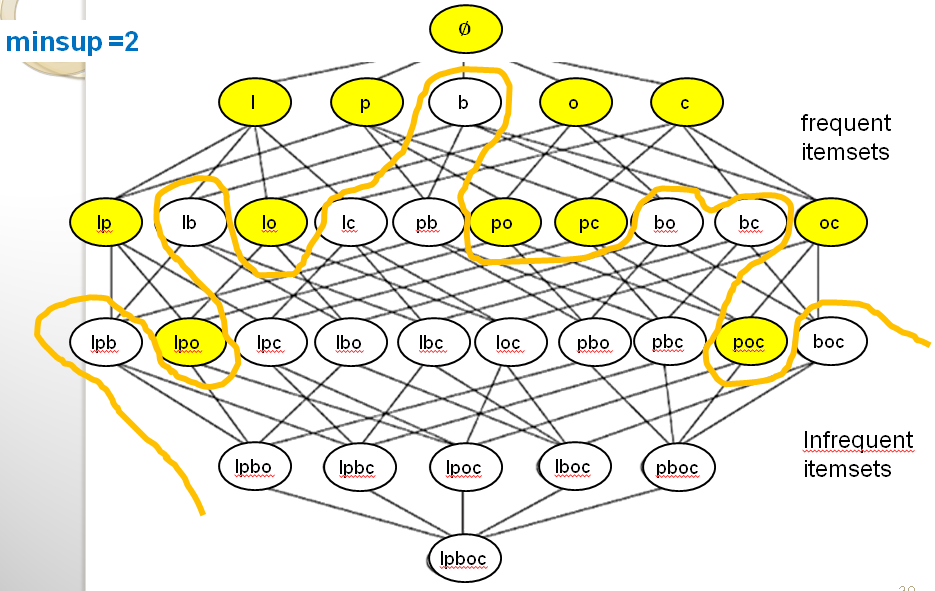In the above picture, we can see that we can draw a line between the frequent itemsets (in yellow) and the infrequent itemsets (in white). This line is drawn based on the fact that all the supersets of an infrequent itemset must also be infrequent due to the Apriori property.  Let me illustrate this more clearly. Consider the itemset {bread} which is infrequent in our example because its support is lower than the minsup threshold. That itemset is shown in red color below.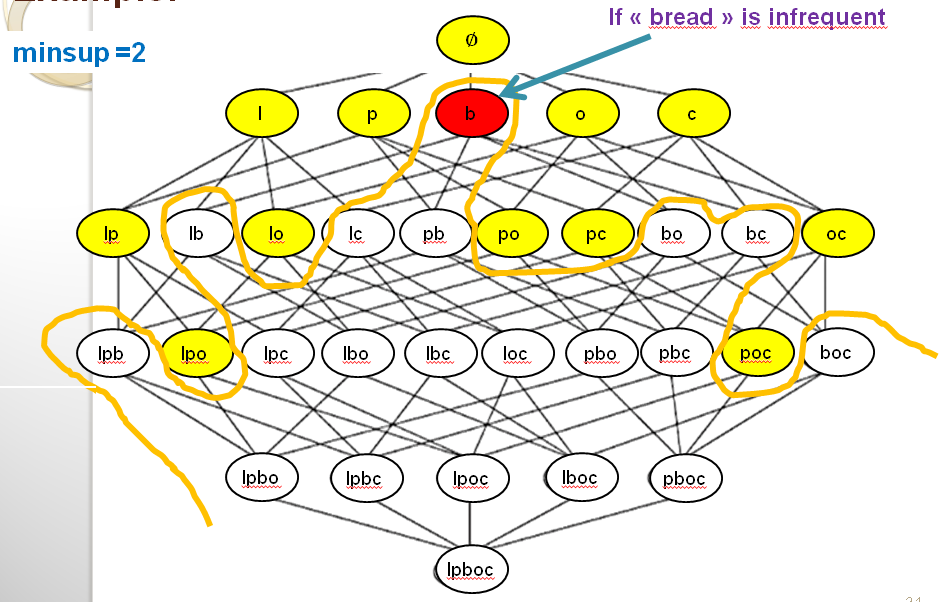Then, based on the Apriori property, because bread is infrequent, all its supersets must be infrequent. Thus we know that any itemset containing bread cannot be a frequent itemset. Below, I have colored all these itemsets in red to make this more clear.Thus, the Apriori property is very powerful.  When an algorithm explores the search space, if it finds that some itemset (e.g. bread) is infrequent, we can avoid considering all itemsets that are supersets of that itemset (e.g. all itemsets containing bread).

A second important property used in the Apriori algorithm is the following.  If an itemset contain a subset that is infrequent, it cannot be a frequent itemset. Let me show an example: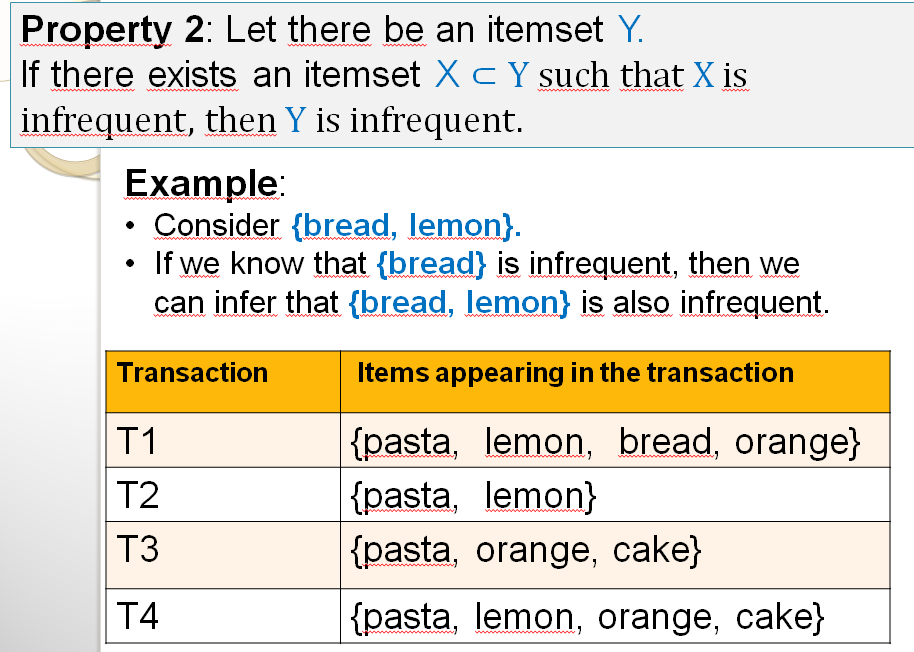The property say that if we have an itemset such as {bread, lemon} that contain a subset that is infrequent such as {bread}, then the itemset cannot be frequent. In our example, since {bread} is infrequent, it means that {bread, lemon} is also infrequent.  You may think that this property is very similar to the first property!  Actually, this is true. It is just a different way of writing the same property. But it will be useful for explaining how the Apriori algorithm works.

The Apriori algorithm

I will now explain how the Apriori algorithm works with an example, as I want to explain it in an intuitive way.  But first, let’s remember what is the input and output of the Apriori algorithm. The input is (1) a transaction database and (2) a minsup threshold set by the user.  The output is the set of frequent itemsets.  For our example, we will consider that minsup = 2 transactions.

The Apriori algorithm is applied as follows. The first step is to scan the database to calculate the support of all items (itemsets containing a single items). The results is shown below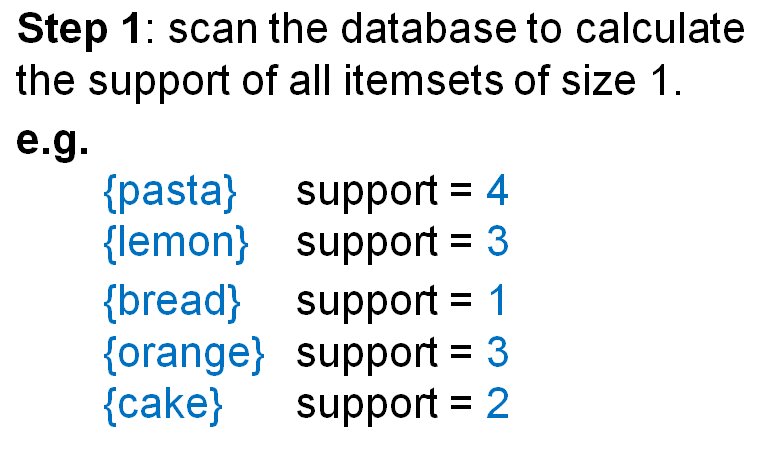After obtaining the support of single items, the second step is to eliminate the infrequent itemsets.  Recall that the minsup parameter is set to 2 in this example. Thus we should eliminate all itemsets having a support that is less than 2. This is illustrated below:We thus now have four itemsets left, which are frequent itemsets. These itemsets are thus output to the user. All these itemsets each contain a single item.

Next the Apriori algorithm will find the frequent itemsets containing 2 items. To do that, the Apriori algorithm combines each frequent itemsets of size 1 (each single item) to obtain a set of candidate itemsets of size 2 (containing 2 items). This is illustrated below: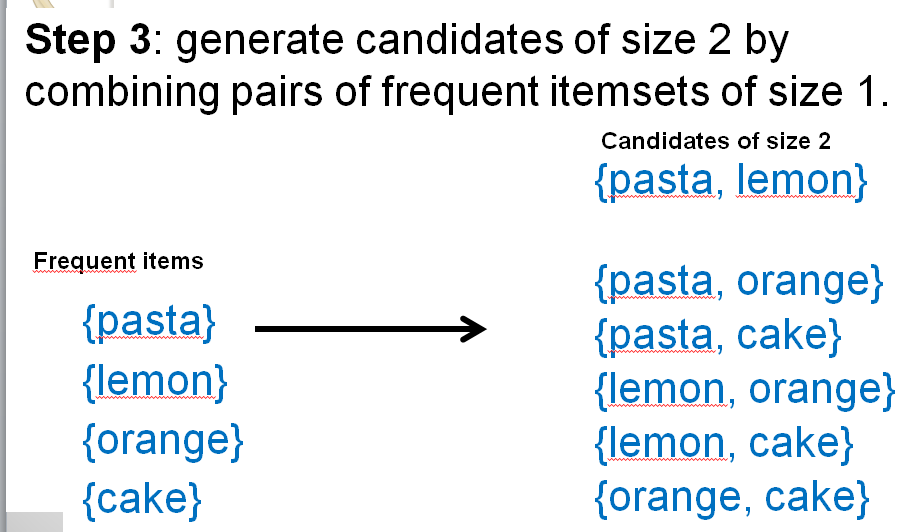Thereafter, Apriori will determine if these candidates are frequent itemsets. This is done by first checking the second property, which says that the subsets of a frequent itemset must also be frequent. For the candidates of size 2, this would be done by checking if the subsets containing 1 items are also frequent. For the candidate itemsets of size 2, it is always true, so the Apriori algorithm does nothing.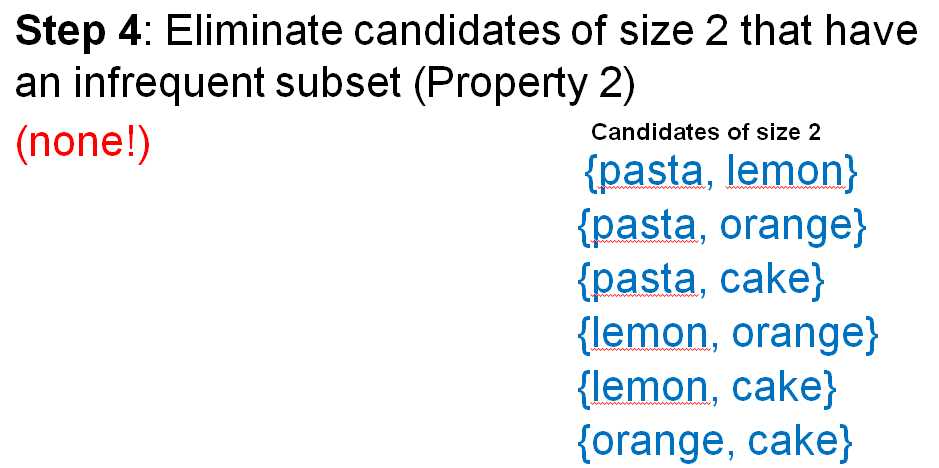Then, the next step is to scan the database to calculate the exact support of the candidate itemsets of size 2, to check if they are reallyfrequent. The result is as follows.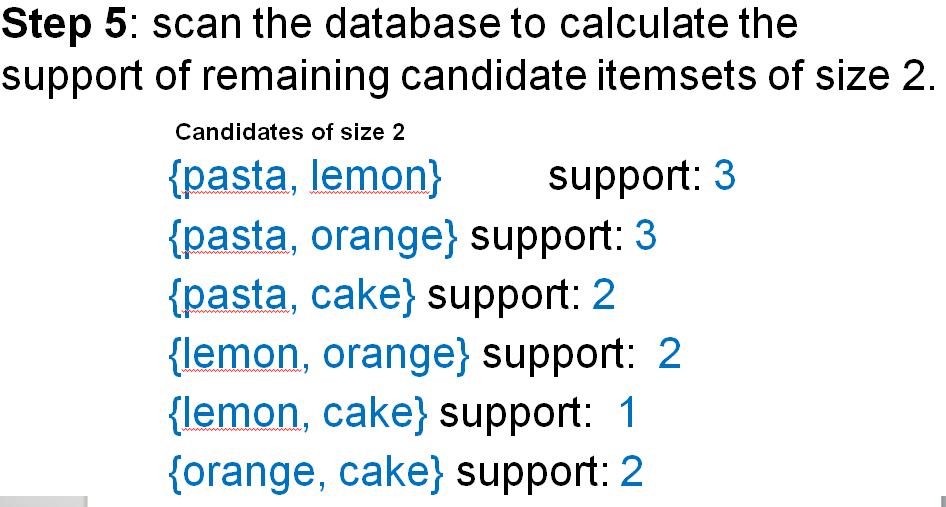Based on these support values,  the Apriori algorithm next eliminates the infrequent candidate itemsets of size 2. The result is shown below: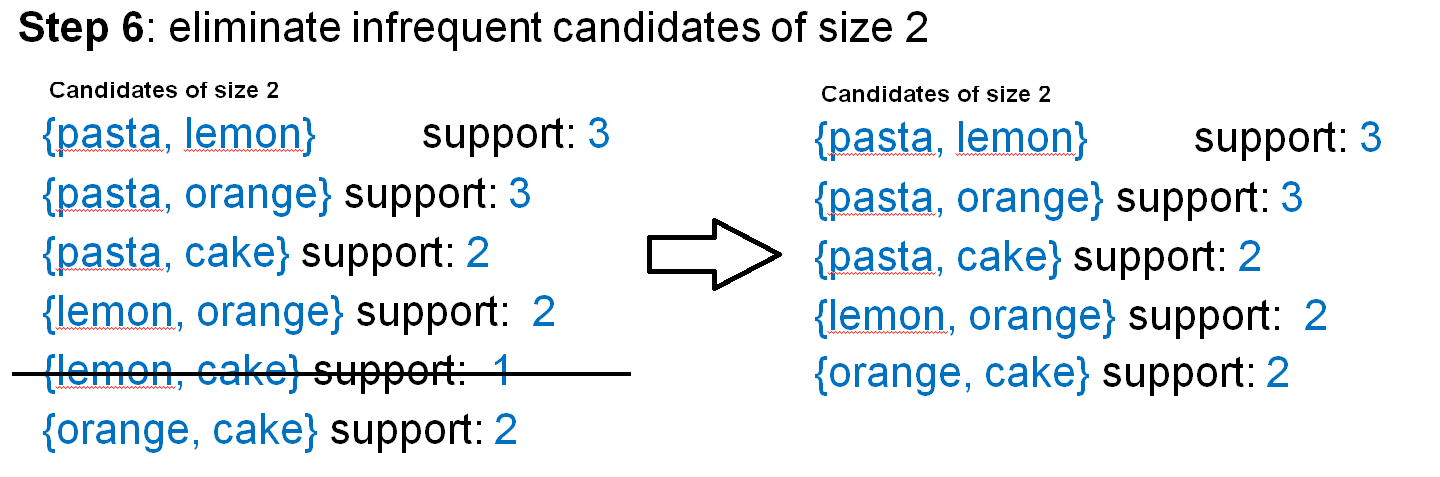As a result, there are only five frequent itemsets left.  The Apriori algorithm will output these itemsets to the user.

Next, the Apriori algorithm will try to generate candidate itemsets of size 3. This is done by combining pairs of frequent itemsets of size 2.  This is done as follows: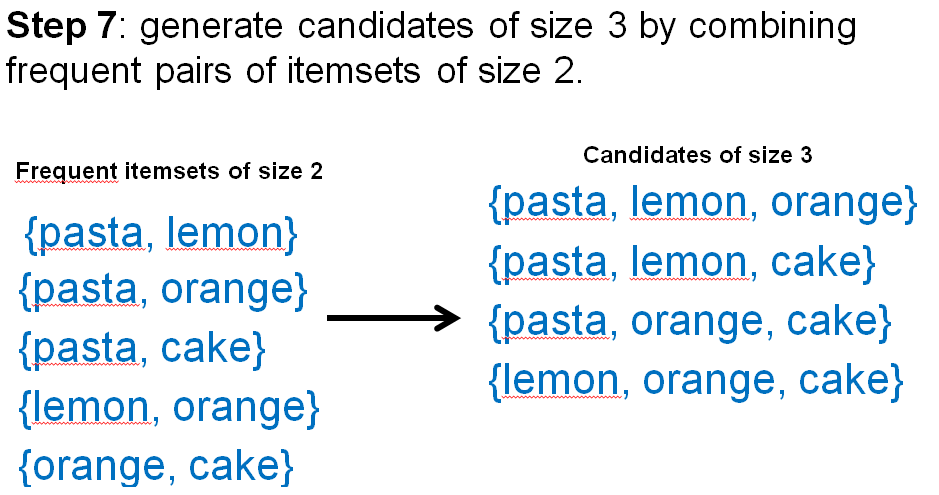Thereafter, Apriori will determine if these candidates are frequent itemsets. This is done by first checking the second property, which says that the subsets of a frequent itemset must also be frequent.  Based on this property, we can eliminate some candidates. The Apriori algorithm checks if there exists a subset of size 2 that is not frequent for each candidate itemset. Two candidates are eliminated as shown below.For example, in the above illustration, the itemset {lemon, orange, cake} has been eliminated because one of its subset of size 2 is infrequent (the itemset {lemon cake}).  Thus, after performing this step, only two candidate itemsets of size 3 are left.

Then, the next step is to scan the database to calculate the exact support of the candidate itemsets of size 3, to check if they are really frequent. The result is as follows.Based on these support values,  the Apriori algorithm next eliminates the infrequent candidate itemsets of size 3 o obtain the frequent itemset of size 3. The result is shown below:There was no infrequent itemsets among the candidate itemsets of size 3, so no itemset was eliminated.  The two candidate itemsets of size 3 are thus frequent and are output to the user.

Next, the Apriori algorithm will try to generate candidate itemsets of size 4. This is done by combining pairs of frequent itemsets of size 3.  This is done as follows: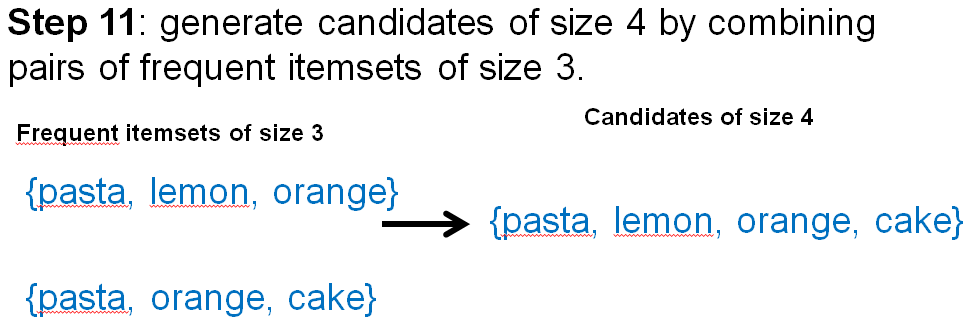Only one candidate itemset was generated. hereafter, Apriori will determine if this candidate is frequent. This is done by first checking the second property, which says that the subsets of a frequent itemset must also be frequent.  The Apriori algorithm checks if there exist a subset of size 3 that is not frequent for the candidate itemset.During the above step,  the candidate itemset {pasta, lemon, orange, cake} is eliminated because it contains at least one subset of size 3 that is infrequent. For example, {pasta, lemon cake} is infrequent.

Now, since there is no more candidate left. The Apriori algorithm has to stop and do not need to consider larger itemsets (for example, itemsets containing five items).

The final result found by the algorithm is this set of frequent itemsets.Thus, the Apriori algorithm has found 11 frequent itemsets. The Apriori algorithm is said to be a recursive algorithm as it recursively explores larger itemsets starting from itemsets of size 1.

Now let’s analyze the performance of the Apriori algorithm for the above example. By using the two pruning properties of the Apriori algorithm, only 18 candidate itemsets have been generated. However, there was 31 posible itemsets that could be formed with the five items of this example (by excluding the empty set). Thus, thanks to its pruning properties the Apriori algorithm avoided considering 13 infrequent itemsets. This may not seems a lot, but for real databases, these pruning properties can make Apriori quite efficient.

It can be proven that the Apriori algorithm is complete (that it will find all frequent itemsets in a given database) and that it is correct (that it will not find any infrequent itemsets). However, I will not show the proof here, as I want to keep this blog post simple.

Technical details

Now, a good question is how to implement the Apriori algorithm. If you want to implement the Apriori algorithm, there are more details that need to be considered.  The most important one is how to combine itemsets of a given size k to generate candidate of a size k+1.

Consider an example.  Let’s say that we combine frequent itemsets containing 2 items to generate candidate itemsets containing 3 items. Consider that we have three itemsets of size 2 : {A,B}, {A,E} and {B,E}.

A problem is that if we combine  {A,B} with {A,E}, we obtain {A,B,E}.  But if we combine {A,E} with {B,E}, we also obtain {A,B,E}. Thus, as shown in this example, if we combine all itemsets of size 2 with all other itemsets of size 2, we may generate the same itemset several times and this will be very inefficient.

There is a simple trick to avoid this problem. It is to sort the items in each itemset according to some order such as the alphabetical order. Then, two itemsets should only be combined if they have all the same items except the last one.

Thus, {A,B} and {A,E}  can be combined since only the last item is different.  But  {B,E} and {A,E} cannot be combined since some items are different that are not the last item of these itemsets. By doing this simple strategy, we can ensure that Apriori will never generate the same itemset more than once.

I will not show the proof to keep this blog post simple. But it is very important to use this strategy when implementing the Apriori algorithm.

How is the performance of the Apriori algorithm?

In general the Apriori algorithm is much faster than a naive approach where we would count the support of all possible itemsets, as Apriori will avoid considering many infrequent itemsets.

The performance of Apriori can be evaluated in real-life in terms of various criteria such as the execution time, the memory consumption, and also its scalability (how the execution time and memory usage vary when increasing the amount of data). Typically, researchers in the field of data mining will perform numerous experiments to evaluate the performance of an algorithm in comparison to other algorithms.  For example, here is a simple experiment that I have done to compare the performance of Apriori with other frequent itemset mining algorithms on a dataset called “Chess“.In that experiment, I have varied the minimum support threshold to see the influence on the execution time of the algorithms. As the threshold is set lower, more patterns need to be considered and the algorithms become slower. It can be seen that Apriori performs quite well but is still much slower than other algorithms such as Eclat and FPGrowth.  This is normal since the Apriori algorithm actually has some limitations that have been addressed in newer algorithms. For example, Apriori is an algorithm that can generate candidate itemsets that do not exist in the database (have a support of 0). More recent algorithms such as FPGrowth are designed to avoid this problem. Besides, note that here, I just show results on a single dataset. To perform a complete performance comparison, we should consider more than a single dataset. But I just show this as an example in this blog post. The experiment shown here was run with the SPMF data mining software which offers open-source implementations of Apriori and many other pattern mining algorithms in Java.

In this blog post, I have aimed at giving a brief introduction to the Apriori algorithm. I did not discuss optimizations, but there are many optimizations that have been proposed to efficiently implement the Apriori algorithm.

If you want to know more about Apriori, you could read the original paper by Agrawal published in 1993:

Rakesh Agrawal and Ramakrishnan Srikant Fast algorithms for mining association rules. Proceedings of the 20th International Conference on Very Large Data Bases, VLDB, pages 487-499, Santiago, Chile, September 1994.

To try Apriori, you can obtain a fast  implementation of Apriori as part of the SPMF data mining software, which is implemented in Java under the GPL3 open-source license.  The source code of Apriori in SPMF is easy to understand, fast, and lightweight (no dependencies to other libraries).

On the website of SPMF, examples and datasets are provided for running the Apriori algorithm, as well as more than 100 other algorithms for pattern mining. The source code of algorithms in SPMF has no dependencies to other libraries and can be easily integrated in other software. The SPMF software also provides a simple user-interface for running algorithms:Besides, if you want to know more about frequent itemset mining, I recommend to read my recent survey paper about itemset mining . It is easy to read and goes beyond what I have discussed in this blog post. The survey paper is more formal, gives pseudocode of Apriori and other algorithms,  and also discusses extensions of the problem of frequent itemset mining and research opportunities.

Hope you have enjoyed this blog post! 😉

Philippe Fournier-Viger is a professor of computer science and founder of the SPMF data mining library.

(Visited 5,419 times, 2 visits today)

### Related posts:

#### Introduction to the Apriori algorithm (with Java code) — 23 Comments

1.James on said:

Thanks for these nice articles. I’m really learning things here.

The least that I can do as a “Thank you” is to write down any (important) typo which I find, to help you make these posts even better.

Here is the first one:
Original: “The support of X must be less than or equal to the support of Y”.
FIXED: “The support of Y must be less than or equal to the support of X”.

-J

• Hi James,

Glad you like the blog and have read a few articles. 🙂 Thank you very much for reporting the typo. It is very useful for other people who will also read the blog after you. If you find any other errors, I will be very happy to fix them!

Thanks,

Philippe

•James on said:

My pleasure! 🙂

2.James on said:

Original: “the support of {pasta,lemon} cannot be less than the support of {pasta}. It must be equal or less than he support of {pasta}.”
FIXED: “the support of {pasta,lemon} cannot be MORE than the support of {pasta}. It must be equal or less than he support of {pasta}.”

3.Greg on said:

Thank you for your simple way of explaining what I am looking for. Up until today I did not even know a name of what I am trying to do. I think that the Apriori algorithm should work for what I am wanting to data mine, so I now have a direction to go in instead of wandering around the net looking for clues.

• Hi Greg, Glad that it is helpful. And thanks for commenting 🙂
Philippe

4.Bolorunduro janet on said:

Thank you very much.Am New in Data mining and i dont know how to run the soft ware i need Assistance.

• If you go to the download page of the SPMF website, there are some clear instructions that explain how to run the software. Please read them carefully and it should work.

5.Ghizlane on said:

Thank you very much for this blog.

I am new too in data mining and i want some help, I want to implement Apriopri with Multicriretia chose.

What is the main propreties to patterns?

6. Pingback: 高效用项集挖掘介绍 | 数据挖掘博客

7.R-ALGO on said:

Very detailed article on Apriori algorithm. Well done!

8.Felipe on said:

When generating k+1 candidate itemsets, say if I have these frequent 2-itemsets {A,B}, {B,C}, {B,D}. Then, the 3-itemsets candidates should be {A,B,C}, {B,C,D}. However, If if follow the rule that two paris should only be combined only if the last item doesn’t match. {A,B} wouldn’t be paired with any other set. Where would {A,B,C} be generated from?
Thanks!

• Hi,
Yes, if you have only {A,B}, {B,C}, {B,D} and you follow the rule, then it is correct that only {B,C,D} will be generated.

To obtain {A,B,C}, you need to also have {A,C}. Let me show you. If you have {A,B}, {A,C}, {B,C} and {B,D}, and you follow the rule, then {A,B,C} and {B,C,D} will be generated.

Hope this helps.

Philippe

•Felipe on said:

You’re right, not sure how I missed that 😡
Appreciate the help!

•Felipe on said:

Wrong emote by the way, should’ve been instead haha!

• Haha… that is fine 😉 Glad it is clear!

9.Renato on said:

Congratulations and thanks for such a great information, I have one question about which algorithm I might use if I have repeated items in a transaction, example: t1:{A,B,C,D,C,B,E}, t1:{A,B,B,C,E}, t1:{A,B,C,E,D} and the are not ordered as you may notice.

• Hi,

10.Peter on said: